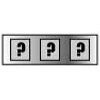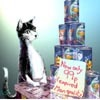#### You may also like### Summing Consecutive Numbers

15 = 7 + 8 and 10 = 1 + 2 + 3 + 4. Can you say which numbers can be expressed as the sum of two or more consecutive integers?### Forgotten Number

I have forgotten the number of the combination of the lock on my briefcase. I did have a method for remembering it...### Human Food

Sam displays cans in 3 triangular stacks. With the same number he could make one large triangular stack or stack them all in a square based pyramid. How many cans are there how were they arranged?

# Handshakes

##### Age 11 to 14Challenge Level

A student from Mearns Castle made the useful connection with another problem published this month:

This is similar to finding the number of lines in Mystic Rose.

Say there are n people. The first person shakes his hand with the other n-1 people.
The second person then shakes his hand with the other n-2 people.
And so on until the (n-1)th person shakes his hand with the nth person.

So the number of handshakes is (n-1) + (n-2)... + 3 + 2 + 1 which equals (n-1)(n)/2.

Another way of considering it is that each person has a total of n-1 handshakes, and there are n people, so there would be (n-1)(n) handshakes, but this includes each handshake twice (1 and 2, 2 and 1) so dividing by 2 gives the correct answer.

Aswaath from the Garden International School in Kuala Lumpur, Malaysia mentioned that the method for solving this problem connected with the method used by Gauss when he was still a young student.

Joe from Hove Park Lower School also noticed connections with other work:

If, for example, 10 mathematicians met, the first will make 9 handshakes, the second makes 8, the third makes 7 and so on until the tenth finds he has already made handshakes with everyone and so makes no more.

This gives 9+8+7+6+5+4+3+2+1+0 handshakes and this is 45.

But look at the sequence... it is the 9th triangular number.
(See Picturing Triangle Numbers and/or Clever Carl)

The formula for the Tth triangular number is T(T+1)/2
With the handshake problem, if there are n people, then the number of handshakes is equivalent to the (n-1)th triangular number.

Subsituting T = n-1 in the formula for triangular numbers, we can deduce a formula for the number of handshakes between n people:

Number of handshakes = (n-1)(n)/2

Jayme from the Garden International School agreed and used this insight to correct Sam's reasoning:

Sam's method isn't right because he did not divide the answer by 2. If you do not divide it by 2, you will be counting the number of handshakes between pairs of mathematicians twice.

If 20 mathematicians meet:
20 x 19 = 380
380 / 2 = 190 (total number of handshakes)

If 161 mathematicians meet:
161 x 160 = 25760
25760 / 2 = 12880 (total number of handshakes)

Could there be exactly 4851 handshakes at a gathering where everyone shakes hands?

We know that 4851 handshakes is between 20 mathematicians and 160 mathematicians. So, let's start by trying out 100 mathematicians.
Total number of handshakes = 100 x 99 / 2 = 4950

That's too many so now I'll try 99 mathematicians.
Total number of handshakes = 99 x 98 / 2 = 4851
Therefore, there could be exactly 4851 handshakes when 99 mathematicians meet.

Could there be exactly 6214 handshakes at a gathering where everyone shakes hands?

Let's start by trying out 112 mathematicians.
Total number of handshakes = 112 x 111/ 2 = 6216

That's too many so now I'll try 111 mathematicians.
Total number of handshakes = 111 x 110 / 2 = 6105
That's too few so there cannot be exactly 6214 handshakes.

Joseph from Bradon Forest School and Tabitha from The Norwood School used similar reasoning:

Sam's reasoning is wrong because you should only count the unique handshakes that have taken place. He should modify his method by dividing by 2. Each handshake involves 2 mathematicians so only half of Sam's handshakes are unique.

$161$ mathematicians
Total handshakes = $161 \times 160/2 = 12880$

Quick Method:
$N$ = number of mathematicians
Total handshakes = $N\times (N - 1)/2$ or $(N^2 -N)/2$

Can $4851$ be the exact number of handshakes at a gathering?
$(N^2 -N)/2 = 4851$
$(N^2 -N)= 9702$

$9702 = approx. 10000 = 100^2$
Try $N = 99$
$99^2 -99 = 97024$

So yes, if $99$ mathematicians meet there will be $4851$ handshakes

$6214$ handshakes?
No, the nearest triangle number is $6216$, and $6214$ is not a triangle number

$3655$ handshakes?
Yes, $86$ Mathematicians

$7626$ handshakes?
Yes, $124$ Mathematicians

$8656$ handshakes?
No, the nearest triangle number is $8646$, and $8656$ is not a triangle number.

We received many more correct solutions, including very clear ones from Siddhartha and Tasuku from the Garden International School in Kuala Lumpur, Ben from Bedminster Down School, Abhinav from Bangkok Patana School and Luke from Maidstone Grammar School. Well done and thank you to you all.